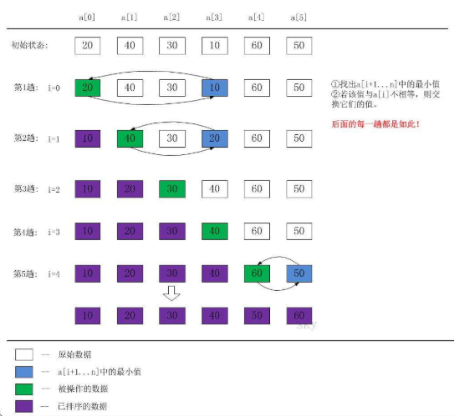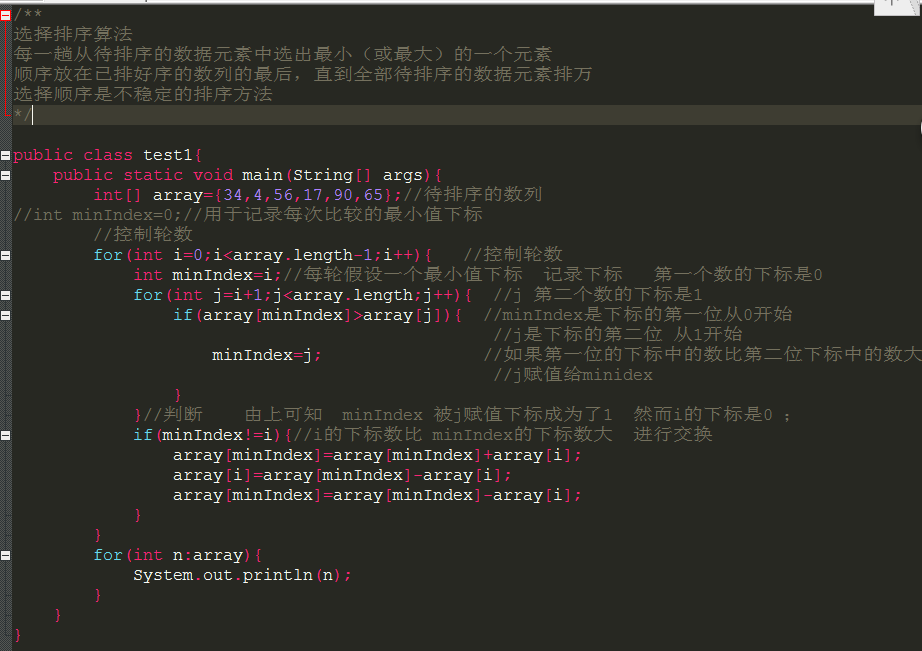• java选择排序
java 选择排序

*************************

选择排序算法描述：原始数组长度n，初始排序数组长度0，未排序数组长度n

从未排序数组中选取最小值，交换到数组开头，此时排序数组长度为1，未排序数组长度n-1；

选取剩余数组中最小值，交换到数组第2位，此时排序数组长度为2，未排序数组长度为n-2；

重复执行上述过程，直至排序数组长度为n

空间复杂度：选择排序需要一个额外空间，空间复杂度为o(1)

时间复杂度：o(n^2)

算法稳定性：想通大小元素的相对位置在排序前后可能会改变，选择排序算法不稳定

*************************

示例

public class MyTest5 {

public static int[] generate(int length){
int[] a=new int[length];

for (int i=0;i<=length-1;i++){
a[i]=new Random().nextInt(1000);
}

return a;
}

public static void selectSort(int[] a){
for (int i=0;i<a.length-1;i++){
int min=i;

for (int j=i+1;j<a.length;j++){
if (a[j]<a[min]){
min=j;
}
}

if (min!=i){
int temp=a[i];
a[i]=a[min];
a[min]=temp;
}
}
}

public static void main(String[] args){
int[] a=generate(10);
System.out.println("数组排序前为：");
Arrays.stream(a).forEach(i -> System.out.print(i+" "));

selectSort(a);
System.out.println("\n\n数组排序后为：");
Arrays.stream(a).forEach(i -> System.out.print(i+" "));
}
}


控制台输出

数组排序前为：
25 738 481 544 212 850 379 684 579 273

数组排序后为：
25 212 273 379 481 544 579 684 738 850



展开全文• ## java选择排序

千次阅读 2017-12-03 20:08:03
java选择排序展开全文• Java选择排序，Selection sort 选择排序与冒泡排序（Bubble Sort）相似，只是冒泡排序再比较之后满足条件就立即交换元素的位置，而选择排序不会立即交换元素的位置，会用一个temp记录下满足条件的元素的下标。当一...
Java选择排序，Selection sort
选择排序与冒泡排序（Bubble Sort）相似，只是冒泡排序再比较之后满足条件就立即交换元素的位置，而选择排序不会立即交换元素的位置，会用一个temp记录下满足条件的元素的下标。当一轮比较结束后，被记录下标的元素与次轮的首元素交换位置。
代码：
public class SelectionSort {public static void main(String[] args) {int[] arr = {2,5,5,7,1,3,9,8,6,10};int minIndex;for(int i=0; i<arr.length; i++){minIndex = i;for(int j=i+1; j<arr.length; j++){if(arr[minIndex]>arr[j]){minIndex=j;}}if(minIndex!=i){int temp = arr[minIndex];arr[minIndex] = arr[i];arr[i] = temp;}}for(int k=0; k<arr.length; k++){System.out.println(arr[k]);}}
}

展开全文• 选择排序 选择排序思想： 从当前位置（每一轮位置不变）开始和后面的较小值进行位置交换 选择排序实现： 控制排序轮数：排序轮数：数组长度-1 控制每轮从当前位置开始比较几次 public static void main(String[] ...
选择排序
选择排序思想：
从当前位置（每一轮位置不变）开始和后面的较小值进行位置交换
选择排序实现：

控制排序轮数：排序轮数：数组长度-1
控制每轮从当前位置开始比较几次

	public static void main(String[] args) {
/**
* 选择排序
*/
int[] arr = { 5, 1, 9, 30, 2, 4 };

for (int i = 0; i < arr.length - 1; i++) {

for (int j = i + 1; j < arr.length; j++) {
if (arr[i] > arr[j]) {
int temp = arr[i];
arr[i] = arr[j];
arr[j] = temp;
}
}

}

System.out.println(Arrays.toString(arr));

}



展开全文算法 数据结构
• 一、选择排序是一种不稳定的排序方法，其主要思想是：每趟排序在当前待排序序列中选出关键码最小的记录，添加到有序序列中。通俗理解即：当前值和后序队列中所有制进行比较，选出最小的。 二、代码理解 class ......

# java选择排序java 订阅